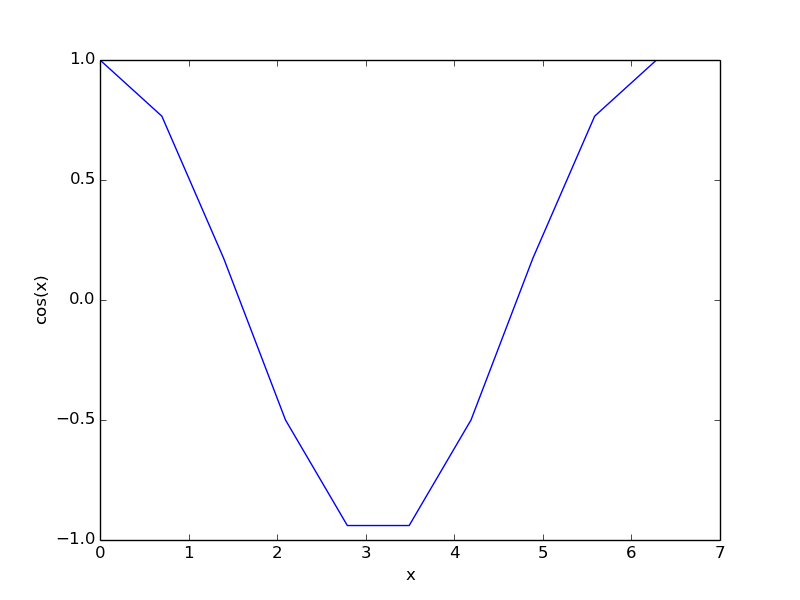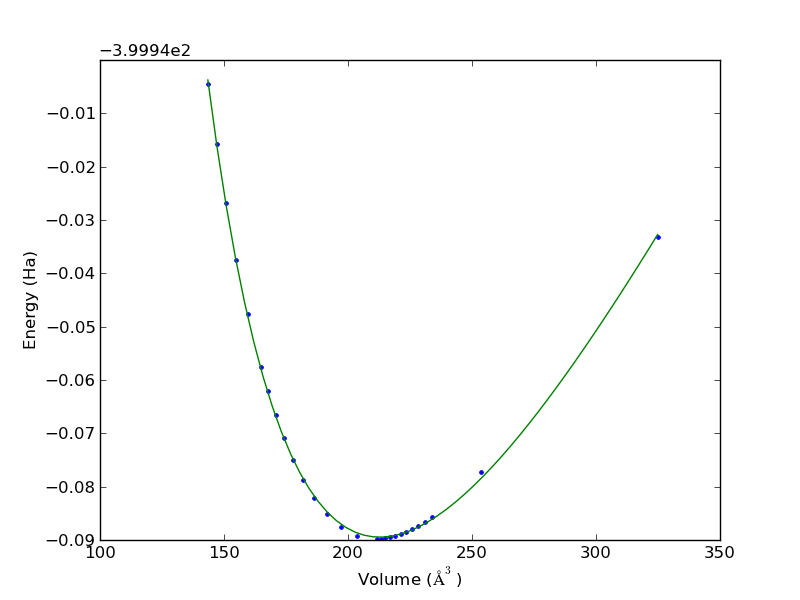Getting a list of figures in an org-buffer

| categories: org-mode | tags: | View Comments

Similar to the previous example of getting a list of tables, here we examine getting a list of figures. Here are two figure links, one with a label, and one with a caption.Figure 1: An equation of state. this is the caption of the figure.Figure 2: another figure

We define a link that will parse the buffer, and create links in a new buffer to the figures. We define a figure as a link with a :type of "file" that has a path that points to a file ending with png or pdf. We will improve on the list of tables by making the buffer read-only, and making a local key binding to kill the buffer by pressing "q". Here is our attempted code.

;; http://www.emacswiki.org/emacs/ElispCookbook#toc4
(defun string/ends-with (s ending)
"return non-nil if string S ends with ENDING."
(cond ((>= (length s) (length ending))
(let ((elength (length ending)))
(string= (substring s (- 0 elength)) ending)))
(t nil)))

"list-of-figures"
(let* ((c-b (buffer-name))
(counter 0)
(list-of-figures
"create a link for to the figure"
(when
(and (string= (org-element-property :type link) "file")
(string-match-p
"[^.]*\\.\$$png\\|jpg\$$\$"
(incf counter)

(parent (car (cdr (org-element-property :parent link))))
(caption (caaar (plist-get parent :caption)))
(name (plist-get parent :name)))
(if caption
(format
"[[elisp:(progn (switch-to-buffer \"%s\")(goto-char %s))][figure %s: %s]] %s\n"
c-b start counter (or name "") caption)
(format
"[[elisp:(progn (switch-to-buffer \"%s\")(goto-char %s))][figure %s: %s]]\n"
c-b start counter (or name "")))))))))
(switch-to-buffer "*List of Figures*")
(org-mode)
(erase-buffer)
(insert (mapconcat 'identity list-of-figures ""))
(use-local-map (copy-keymap org-mode-map))
(local-set-key "q" #'(lambda () (interactive) (kill-buffer)))))
(lambda (keyword desc format)
(cond
((eq format 'latex)
(format "\\listoffigures")))))


This is a test to see if our function works for other image types. smiley.jpg

And a link to test it out:

This works too. I am not sure I am getting the figure name and caption in a bulletproof way. They seem to be buried in the :parent of the element, which is a paragraph element. The caption seems to be buried in a few sets of parentheses, hence the use of caaar to get the caption out. I am not sure if the caption is always at that depth or not. As a proof of concept though, this is not too bad.